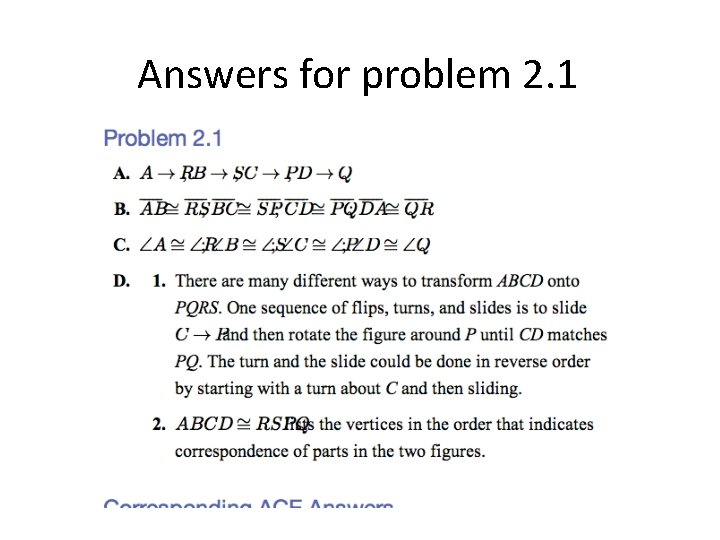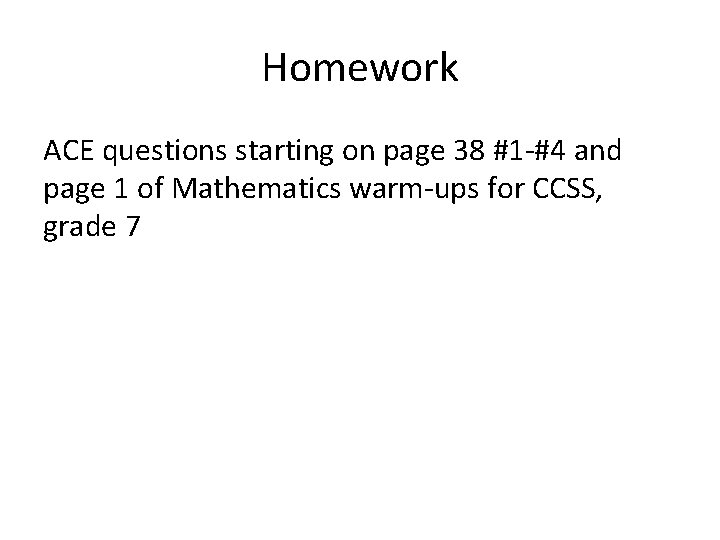# Linear Algebra Monday August 25 Things to do

• Slides: 14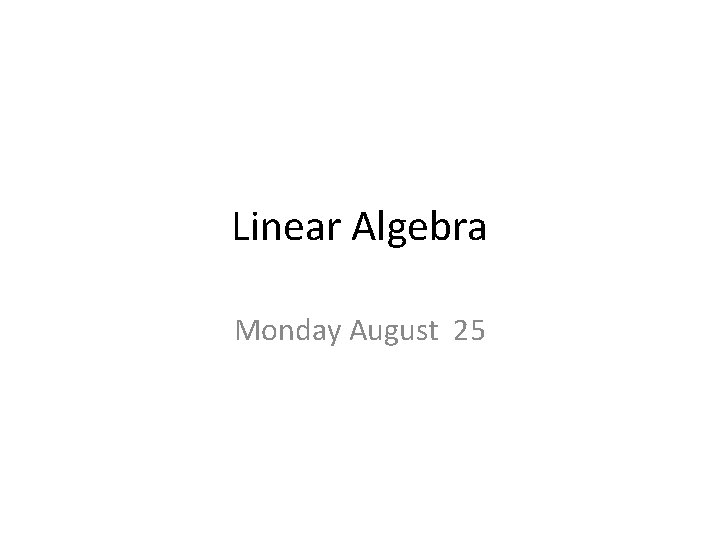Linear Algebra Monday August 25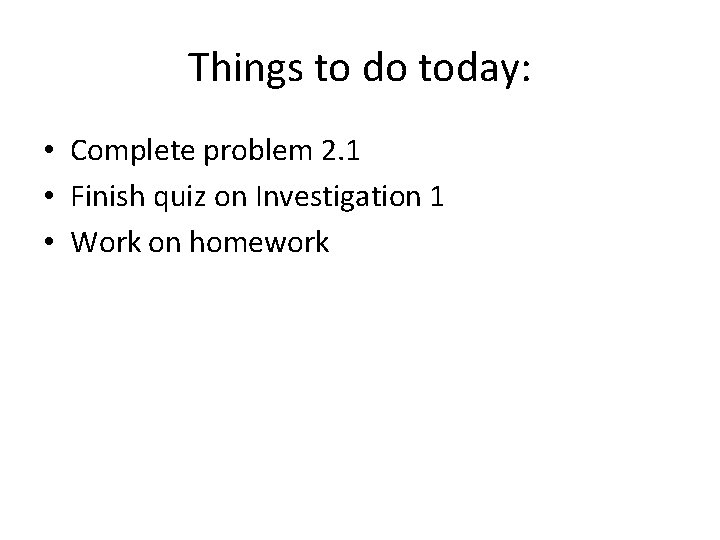Things to do today: • Complete problem 2. 1 • Finish quiz on Investigation 1 • Work on homework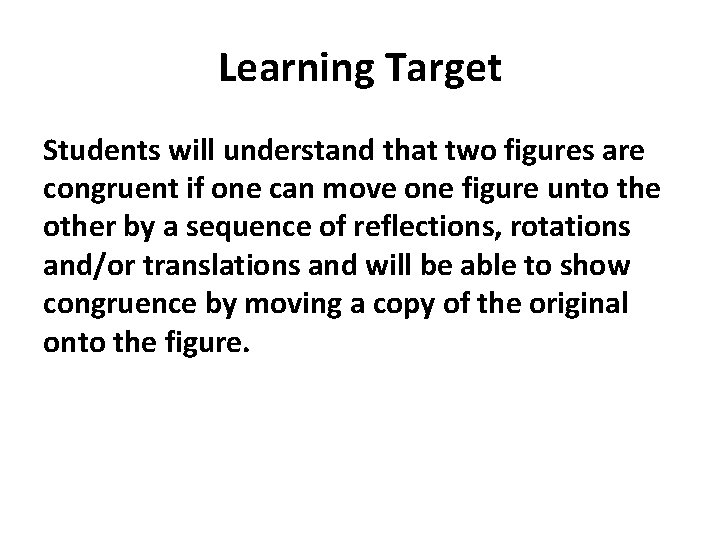Learning Target Students will understand that two figures are congruent if one can move one figure unto the other by a sequence of reflections, rotations and/or translations and will be able to show congruence by moving a copy of the original onto the figure.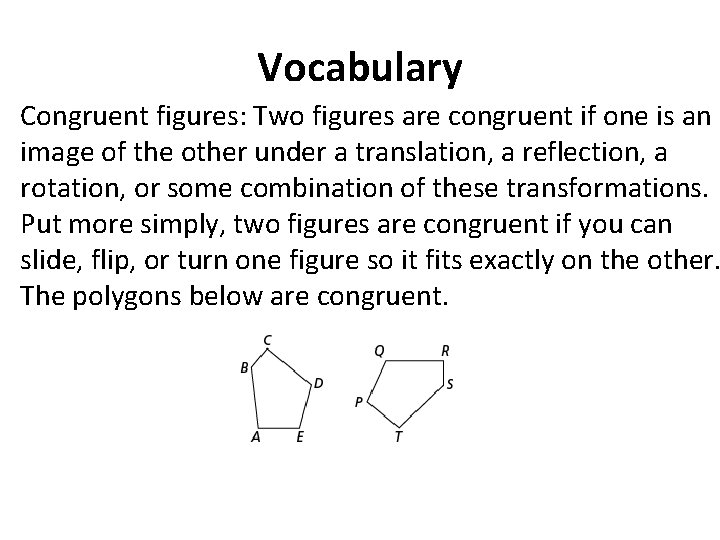Vocabulary Congruent figures: Two figures are congruent if one is an image of the other under a translation, a reflection, a rotation, or some combination of these transformations. Put more simply, two figures are congruent if you can slide, flip, or turn one figure so it fits exactly on the other. The polygons below are congruent.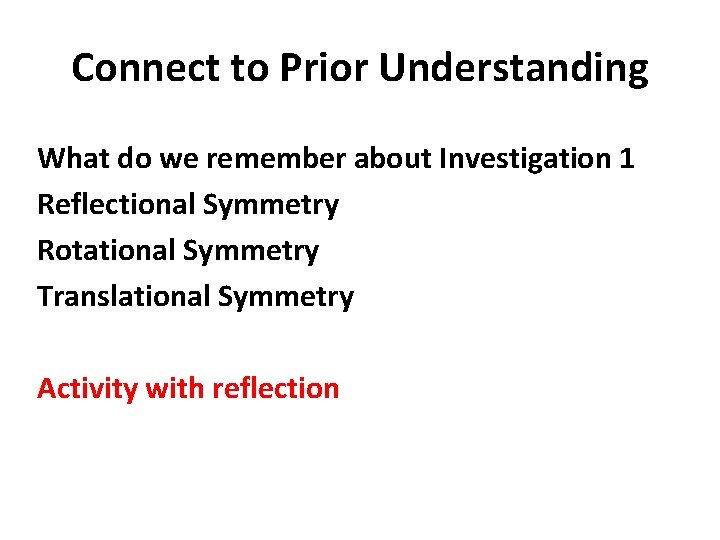Connect to Prior Understanding What do we remember about Investigation 1 Reflectional Symmetry Rotational Symmetry Translational Symmetry Activity with reflection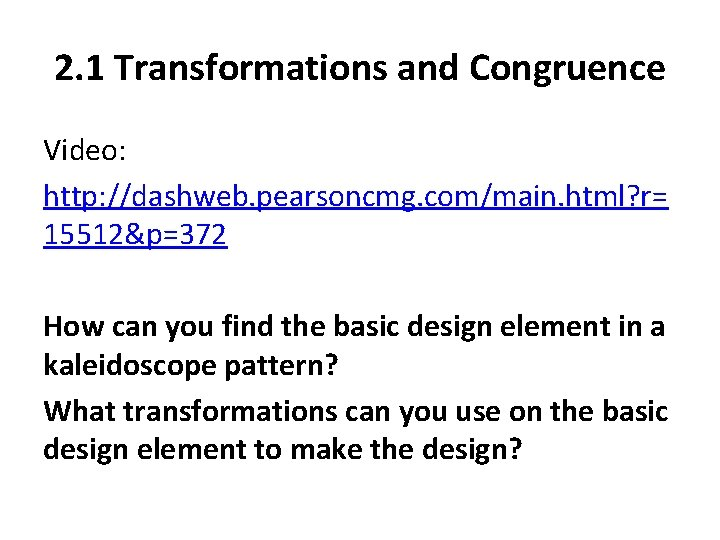2. 1 Transformations and Congruence Video: http: //dashweb. pearsoncmg. com/main. html? r= 15512&p=372 How can you find the basic design element in a kaleidoscope pattern? What transformations can you use on the basic design element to make the design?Kaleidoscopes What transformation strategies could be used to replicate the basic design element and complete the full figure?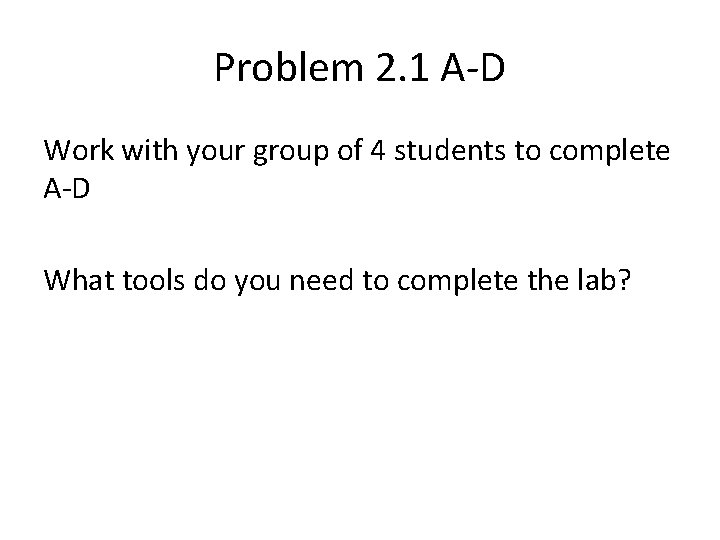Problem 2. 1 A-D Work with your group of 4 students to complete A-D What tools do you need to complete the lab?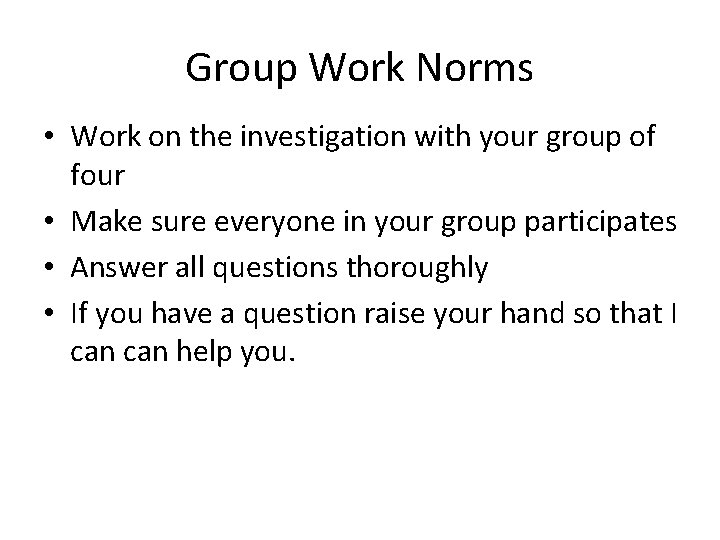Group Work Norms • Work on the investigation with your group of four • Make sure everyone in your group participates • Answer all questions thoroughly • If you have a question raise your hand so that I can help you.Problem 2. 1 When two polygons are congruent you can match the vertices in a way that pairs sides and angles of the same size. What are the pairs of congruent sides and angles? How can you flip, turn and or slide ABCD onto PQRS?Share answers • Who started with a slide? • Did anyone start by rotating the polygon? If you did, how did you find the center and angle of rotation? • What do you mean when you say side RS corresponds to side AB or angle S corresponds to angle B. • Can you say that side RS corresponds to side BA?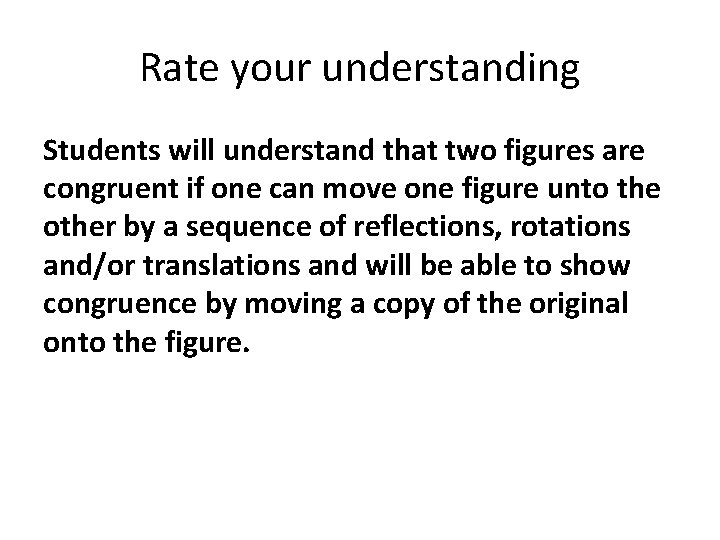Rate your understanding Students will understand that two figures are congruent if one can move one figure unto the other by a sequence of reflections, rotations and/or translations and will be able to show congruence by moving a copy of the original onto the figure.